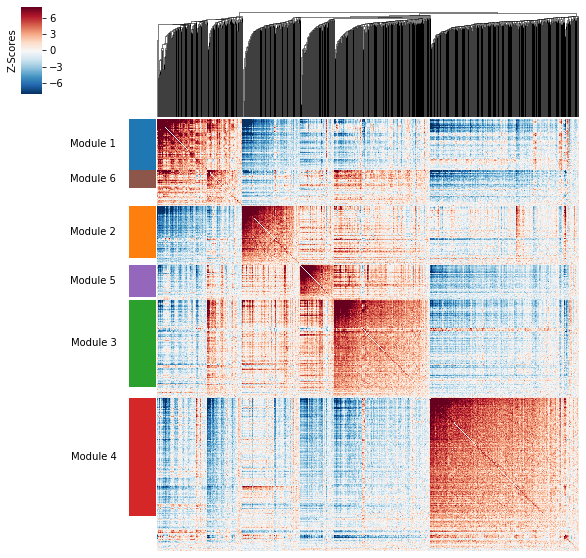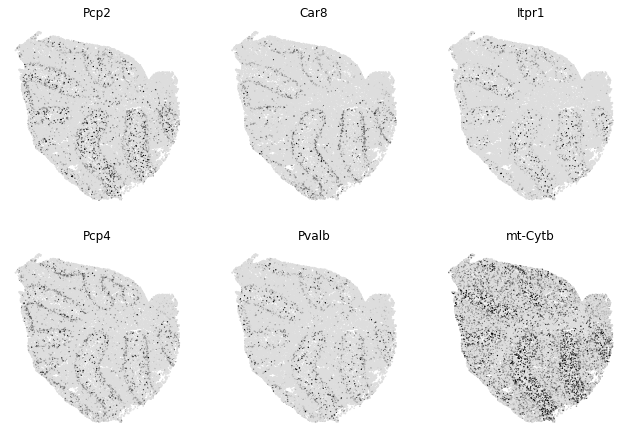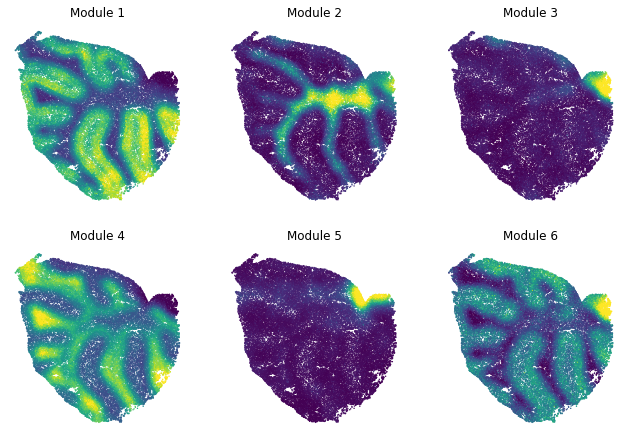# Demo: Spatial data from Slide-seq¶

This demo uses data from:

Slide-seq: A scalable technology for measuring genome-wide expression at high spatial resolution

For this demo, you need the data files for Puck_180819_12

Specifically these two files:

• MappedDGEForR.csv

## Covered Here¶

• Data preprocessing and filtering
• Computing Autocorrelation in Hotspot to identify spatial genes
• Computing local correlations between spatial genes to identify modules
• Plotting modules, correlations, and module scores
:

import numpy as np
import pandas as pd
import hotspot
import matplotlib.pyplot as plt
import matplotlib.colors
import seaborn as sns

# Load the counts and positions
counts_file = 'MappedDGEForR.csv'

counts = pd.read_csv(counts_file, index_col=0) # Takes a while, ~10min

# Align the indices
counts = counts.loc[:, pos.index]
barcodes = pos.index.values

# Swap position axes
# We swap x'=y and y'=-x to match the slides in the paper
pos = pd.DataFrame(
{
'X': pos.ycoord,
'Y': pos.xcoord*-1,
}, index=pos.index
)

num_umi = counts.sum(axis=0)

# Filter genes
gene_counts = (counts > 0).sum(axis=1)
valid_genes = gene_counts >= 50
counts = counts.loc[valid_genes]


## Creating the Hotspot object¶

To start an analysis, first creat the hotspot object When creating the object, you need to specify:

• The gene counts matrix
• Which background model to use
• The latent space we are using to compute our cell metric
• Here we use the physical barcode positions
• The per-cell scaling factor
• Here we use the number of umi per barcode

Once the object is created, the neighborhood graph must be computed with create_knn_graph

The two options that are specificied are n_neighbors which determines the size of the neighborhood, and weighted_graph.

Here we set weighted_graph=False to just use binary, 0-1 weights and n_neighbors=300 to create a local neighborhood size of the nearest 300 barcodes. Larger neighborhood sizes can result in more robust detection of correlations and autocorrelations at a cost of missing more fine-grained, smaller-scale, spatial patterns

:

# Create the Hotspot object and the neighborhood graph

hs = hotspot.Hotspot(counts, model='bernoulli', latent=pos, umi_counts=num_umi)

hs.create_knn_graph(
weighted_graph=False, n_neighbors=300,
)


## Determining genes with spatial variation¶

Now we compute autocorrelations for each gene, using the spatial metric, to determine which genes have the most spatial variation.

:

hs_results = hs.compute_autocorrelations(jobs=16)


100%|██████████| 6942/6942 [00:27<00:00, 250.89it/s]

:

G EG stdG Z C Pval FDR
Gene
Ttr 1.895617e+06 0 4349.885976 435.785413 0.195658 0.0 0.0
Plp1 1.070512e+06 0 4349.885976 246.101150 0.109810 0.0 0.0
Enpp2 9.047060e+05 0 4349.885976 207.983833 0.099028 0.0 0.0
Fth1 9.041625e+05 0 4349.885976 207.858890 0.092616 0.0 0.0
Cartpt 7.867150e+05 0 4349.885976 180.858760 0.084189 0.0 0.0

## Grouping genes into spatial modules¶

To get a better idea of what spatial patterns exist, it is helpful to group the genes into modules.

Hotspot does this using the concept of “local correlations” - that is, correlations that are computed between genes between cells in the same neighborhood.

Here we avoid running the calculation for all Genes x Genes pairs and instead only run this on genes that have significant spatial autocorrelation to begin with.

The method compute_local_correlations returns a Genes x Genes matrix of Z-scores for the significance of the correlation between genes. This object is also retained in the hs object and is used in the subsequent steps.

:

# Select the genes with significant spatial autocorrelation
hs_genes = hs_results.index[hs_results.FDR < 0.05]

# Compute pair-wise local correlations between these genes
lcz = hs.compute_local_correlations(hs_genes, jobs=20)


  0%|          | 0/845 [00:00<?, ?it/s]

Computing pair-wise local correlation on 845 features...

100%|██████████| 845/845 [00:05<00:00, 157.16it/s]
100%|██████████| 356590/356590 [59:29<00:00, 99.89it/s]


Now that pair-wise local correlations are calculated, we can group genes into modules.

To do this, a convenience method is included create_modules which performs agglomerative clustering with two caveats:

• If the FDR-adjusted p-value of the correlation between two branches exceeds fdr_threshold, then the branches are not merged.
• If two branches are two be merged and they are both have at least min_gene_threshold genes, then the branches are not merged. Further genes that would join to the resulting merged module (and are therefore ambiguous) either remain unassigned (if core_only=True) or are assigned to the module with the smaller average correlations between genes, i.e. the least-dense module (if core_only=False)

The output is a Series that maps gene to module number. Unassigned genes are indicated with a module number of -1

This method was used to preserved substructure (nested modules) while still giving the analyst some control. However, since there are a lot of ways to do hierarchical clustering, you can also manually cluster using the gene-distances in hs.local_correlation_z

:

modules = hs.create_modules(
min_gene_threshold=20, core_only=False, fdr_threshold=0.05
)

modules.value_counts()

:

 4    230
3    170
-1    143
2    102
1    101
5     63
6     36
Name: Module, dtype: int64


## Plotting module correlations¶

A convenience method is supplied to plot the results of hs.create_modules

:

hs.plot_local_correlations()To explore individual genes, we can look at the genes with the top autocorrelation in a given module as these are likely the most informative.

:

# Show the top genes for a module

module = 1

results = hs.results.join(hs.modules)
results = results.loc[results.Module == module]

:

G EG stdG Z C Pval FDR Module
Gene
Pcp2 454045.514481 0 4349.885976 104.381015 0.046222 0.0 0.0 1.0
Car8 384470.481798 0 4349.885976 88.386336 0.039361 0.0 0.0 1.0
Itpr1 309992.054843 0 4349.885976 71.264409 0.031606 0.0 0.0 1.0
Pcp4 287016.421190 0 4349.885976 65.982516 0.029496 0.0 0.0 1.0
Pvalb 277574.727119 0 4349.885976 63.811955 0.028504 0.0 0.0 1.0
mt-Cytb 251459.913992 0 4349.885976 57.808392 0.025916 0.0 0.0 1.0
Gpm6b 229566.984067 0 4349.885976 52.775403 0.023639 0.0 0.0 1.0
mt-Nd4 226437.069373 0 4349.885976 52.055863 0.023182 0.0 0.0 1.0
Calb1 198838.797756 0 4349.885976 45.711267 0.020217 0.0 0.0 1.0
Aldoc 187057.748608 0 4349.885976 43.002909 0.019332 0.0 0.0 1.0

To get an idea of what spatial pattern the module is referencing, we can plot the top module genes’ expression onto the spatial positions

:

# Plot the module scores on top of positions

module = 1

results = hs.results.join(hs.modules)
results = results.loc[results.Module == module]

fig, axs = plt.subplots(2, 3, figsize=(11, 7.5))

cmap = matplotlib.colors.LinearSegmentedColormap.from_list(
'grays', ['#DDDDDD', '#000000'])

for ax, gene in zip(axs.ravel(), genes):

expression = np.log2(hs.counts.loc[gene]/hs.umi_counts*45 + 1) # log-counts per 45 (median UMI/barcode)

vmin = 0
vmax = np.percentile(expression, 95)
vmax = 2

plt.sca(ax)
plt.scatter(x=hs.latent.iloc[:, 0],
y=hs.latent.iloc[:, 1],
s=2,
c=expression,
vmin=vmin,
vmax=vmax,
edgecolors='none',
cmap=cmap
)

for sp in ax.spines.values():
sp.set_visible(False)

plt.xticks([])
plt.yticks([])
plt.title(gene)## Summary Module Scores¶

To aid in the recognition of the general behavior of a module, Hotspot can compute aggregate module scores.

:

module_scores = hs.calculate_module_scores()


  0%|          | 0/6 [00:00<?, ?it/s]

Computing scores for 6 modules...

100%|██████████| 6/6 [01:30<00:00, 12.98s/it]

:

1 2 3 4 5 6
barcodes
CGTACAATTTTTTT 0.822729 -0.443706 -0.094899 -0.414559 -0.198448 0.180391
TTCGTTATTTTTTT 1.232227 -0.421417 -0.254999 -0.472261 -0.213982 0.299108
CAAACCAACCCCCC 0.607751 -0.312146 -0.166309 0.234819 -0.328414 0.367822
TCTTTTCACCCCCC 1.286320 -0.491108 -0.053740 -0.736092 -0.096152 0.377854
GGATTGAACCCCCC 0.133101 -0.544696 -0.157040 -0.326436 -0.133297 0.334153

Here we can visualize these module scores by plotting them over the barcode positions:

:

# Plot the module scores on top of positions

fig, axs = plt.subplots(2, 3, figsize=(11, 7.5))

for ax, module in zip(axs.ravel(), range(1, hs.modules.max()+1)):
scores = hs.module_scores[module]

vmin = np.percentile(scores, 1)
vmax = np.percentile(scores, 99)

plt.sca(ax)
plt.scatter(x=hs.latent.iloc[:, 0],
y=hs.latent.iloc[:, 1],
s=2,
c=scores,
vmin=vmin,
vmax=vmax,
edgecolors='none'
)

for sp in ax.spines.values():
sp.set_visible(False)

plt.xticks([])
plt.yticks([])
plt.title('Module {}'.format(module))